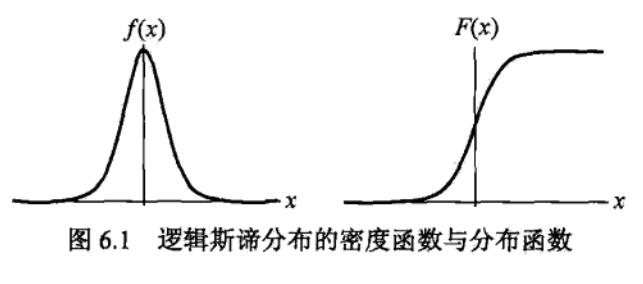# 李航-统计学习方法-笔记-6：逻辑斯谛回归与最大熵模型

## 逻辑斯谛回归

$F(x) = P( X \leqslant x) = \frac{1}{1 + e^{-(x-\mu)/\gamma}}$

$f(x) = F'(x)= \frac{e^{-(x-\mu)/\gamma}}{\gamma (1 + e^{-(x-\mu)/\gamma})^2}$$$F(x)$$属于logistic函数，其图形是一条S形曲线，该曲线以点$$(\mu, \frac{1}{2})$$为中心对称。

$F(\mu+x) + F(\mu-x) = 2 \times \frac{1}{2}$

$$\mu=0, \gamma=1$$时，$$F(x)$$变成sigmoid函数。

$P(Y=1 \ | \ x) = \frac{exp(w \cdot x)}{1 + exp(w \cdot x)}$

$P(Y=0 \ | \ x) = \frac{1}{1 + exp(w \cdot x)}$

logistic回归比较两个条件概率值的大小，将实例$$x$$分到概率值较大的那一类。

$\log \frac{P(Y=1 \ | \ x)}{1 - P(Y=1 \ | \ x)} = w \cdot x$

$P(Y=1 \ | \ x) = \frac{exp(w \cdot x)}{1 + exp(w \cdot x)}$

$$P(Y = 1 \ | \ x) = \pi(x), P(Y = 0 \ | \ x) = 1 - \pi(x)$$

（将上式第一行取反，即为交叉熵损失函数）

$$L(w)$$求极大，得到$$w$$的估计值$$\hat{w}$$。问题变成以对数似然函数为目标的最优化问题，logistic回归学习中通常采用梯度下降法及拟牛顿法。

$P(Y = k \ | \ x) = \frac{exp(w_k \cdot x)}{1 + \sum_{k=1}^{K-1}exp(w_k \cdot x)}, k = 1, 2, ..., K-1$

$P(Y = K \ | \ x) = \frac{1}{1 + \sum_{k=1}^{K-1}exp(w_k \cdot x)}$

## 最大熵模型

$H(P) = - \sum_{x} P(x) \log P(x), \\ 0 \leqslant H(P) \leqslant \log |X|$

$$|X|$$$$X$$的取值个数，当且仅当$$X$$是均匀分布时，不等式右边的等号成立，熵最大。

$\tilde{P}(X = x, Y = y) = \frac{v(X=x, Y=y)}{N}$

$\tilde{P}(X = x) = \frac{v(X=x)}{N}$

$f(x, y) = \left\{\begin{matrix} 1, & x与y满足某一事实\\ 0, & 否则 \end{matrix}\right.$

$E_{\tilde{P}}(f) = \sum_{x, y} \tilde{P}(x, y) \ f(x, y)$

$E_P(f) = \sum_{x, y} \tilde{P}(x) \ P(y \ | \ x) \ f(x, y)$

$$E_P(f) = E_{\tilde{P}}(f), \tag{6.10}$$

$\sum_{x, y} \tilde{P}(x) \ P(y \ | \ x) \ f(x, y) = \sum_{x, y} \tilde{P}(x, y) \ f(x, y), \tag{6.11}$

$\mathcal{C} \equiv \{P \in \mathcal{P} \ | \ E_P(f_i) = E_{\tilde{P}}(f_i), i = 1, 2, ..., n\}$

$H(P) = - \sum_{x, y} \tilde{P}(x) \ P( y \ | \ x) \log P(y \ | \ x)$

## 最大熵模型的学习

$\max_{P \in \mathcal{C}} H(P) = - \sum_{x, y} \tilde{P}(x) \ P( y \ | \ x) \log P(y \ | \ x)$

$\begin{split} s.t. \ \ & E_P(f_i) = E_{\tilde{P}} (f_i), \ \ i = 1, 2, ..., n \\ & \sum_y P( y \ | \ x) = 1\end{split}$

$\min_{P \in \mathcal{C}} - H(P) = \sum_{x, y} \tilde{P}(x) \ P( y \ | \ x) \log P(y \ | \ x)$

$\begin{split} s.t. \ \ & E_P(f_i) - E_{\tilde{P}} (f_i) = 0, \ \ i = 1, 2, ..., n \\ & \sum_y P( y \ | \ x) = 1\end{split}$

$\begin{split} L(P, w) = &- H(P) + w_0 (1 - \sum_y P( y \ | \ x)) +\\ & \sum_{i=1}^{n} w_i (E_P(f_i) - E_{\tilde{P}} (f_i)) \end{split} \tag{6.17}$

$\min_{P \in \mathcal{C}} \max_{w} L(P, w), \tag{6.18}$

$\max_{w} \min_{P \in \mathcal{C}} L(P, w), \tag{6.19}$

$\Psi(w) = \min_{P \in \mathcal{C}} L(P, w) = L(P_w, w), \tag{6.20}$

$$\Psi(w)$$称为对偶函数，同时，将其解记作

$P_w = \arg \min_{P \in \mathcal{C}} L(P, w) = P_w( y \ | \ x)$

$P( y \ | \ x) = exp(\sum_{i=1}^{n} w_i \ f_i(x, y) + w_0 - 1) = \frac{exp(\sum_{i=1}^{n} w_i \ f_i(x, y))}{exp( w_0 - 1)}$

$P_w( y \ | \ x) = \frac{exp(\sum_{i=1}^{n} w_i \ f_i(x, y))}{Z_w(x)}, \tag{6.22}$

$Z_w(x) = \sum_y exp(\sum_{i=1}^{n} w_i \ f_i(x, y)), \tag{6.23}$

## 极大似然估计

$L_{\tilde{P}}(P_w) = \log \prod_{x, y} P( y \ | \ x)^{\tilde{P}(x, y)} = \sum_{x, y} \tilde{P}(x, y) \log P( y \ | \ x)$

$\begin{split}L_{\tilde{P}}(P_w) &= \sum_{x, y} \tilde{P}(x, y) \log P( y \ | \ x) \\ &= \sum_{x, y} \tilde{P}(x, y) \sum_{i=1}^{n} w_i \ f_i(x, y) - \sum_{x, y} \tilde{P}(x, y) \log Z_w(x) \\ &= \sum_{x, y} \tilde{P}(x, y) \sum_{i=1}^{n} w_i \ f_i(x, y) - \sum_{x} \tilde{P}(x) \log Z_w(x)\end{split}$

$\begin{split} \Psi(w) =& \sum_{x, y} \tilde{P}(x) \ P_w( y \ | \ x) \log P_w(y \ | \ x) \\ &+ \sum_{i=1}^{n} w_i (\sum_{x, y} \tilde{P}(x, y) \ f_i(x, y) - \sum_{x, y} \tilde{P}(x) \ P_w( y \ | \ x) \ f_i(x, y)) \\ =& \sum_{x, y} \tilde{P}(x, y) \sum_{i=1}^{n} w_i \ f_i(x, y) + \sum_{x, y} \tilde{P}(x) \ P_w( y \ | \ x) (\log P_w(y \ | \ x) - \sum_{i=1}^{n} w_i f_i(x, y)) \\ =& \sum_{x, y} \tilde{P}(x, y) \sum_{i=1}^{n} w_i \ f_i(x, y) - \sum_{x, y} \tilde{P}(x) \ P_w( y \ | \ x) \log Z_w(x) \\ =& \sum_{x, y} \tilde{P}(x, y) \sum_{i=1}^{n} w_i \ f_i(x, y) - \sum_{x} \tilde{P}(x) \log Z_w(x) \\ \end{split}$

$P_w( y \ | \ x) = \frac{exp(\sum_{i=1}^{n} w_i \ f_i(x, y))}{Z_w(x)}$

$Z_w(x) = \sum_y exp(\sum_{i=1}^{n} w_i \ f_i(x, y))$

## 模型学习的最优化算法

logistic回归模型，最大熵模型的学习以似然函数为目标函数的最优化问题，通常通过迭代算法求解。从最优化观点看，这时的目标函数具有很好的性质，它是光滑的凸函数，因此多种最优化方法都使用，保证能找到全局最优解。

posted @ 2019-06-05 15:39  PilgrimHui  阅读(1532)  评论(0编辑  收藏  举报